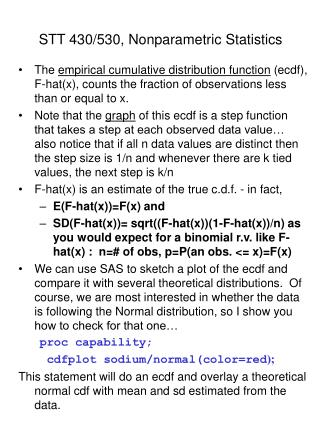DownloadDownload PresentationSTT 430/530, Nonparametric Statistics

# STT 430/530, Nonparametric Statistics

Download Presentation## STT 430/530, Nonparametric Statistics

- - - - - - - - - - - - - - - - - - - - - - - - - - - E N D - - - - - - - - - - - - - - - - - - - - - - - - - - -
##### Presentation Transcript

1. STT 430/530, Nonparametric Statistics • The empirical cumulative distribution function (ecdf), F-hat(x), counts the fraction of observations less than or equal to x. • Note that the graph of this ecdf is a step function that takes a step at each observed data value… also notice that if all n data values are distinct then the step size is 1/n and whenever there are k tied values, the next step is k/n • F-hat(x) is an estimate of the true c.d.f. - in fact, • E(F-hat(x))=F(x) and • SD(F-hat(x))= sqrt((F-hat(x))(1-F-hat(x))/n) as you would expect for a binomial r.v. like F-hat(x) : n=# of obs, p=P(an obs. <= x)=F(x) • We can use SAS to sketch a plot of the ecdf and compare it with several theoretical distributions. Of course, we are most interested in whether the data is following the Normal distribution, so I show you how to check for that one… proccapability; cdfplot sodium/normal(color=red); This statement will do an ecdf and overlay a theoretical normal cdf with mean and sd estimated from the data.

2. Another important graph for checking normality of data is called a normal quantile plot . This plots the sorted data values against the corresponding normal quantile. That is, • first, sort the data from smallest to largest • second, for each data point find the ecdf (i.e., the fraction of the data <= that point) • third, get the corresponding standard normal z-score for that fraction. • Try this SAS code to check it out (recall that the sodium values are already sorted from smallest to largest; if they weren't, then you'd have to use PROC SORT and OUTPUT the sorted data to a SAS data set…: fract=_n_/40; z=probit(fract); probit is the SAS function that returns the z-score corresponding to the cumulative probability under the standard normal curve between 0 and 1. • PROC UNIVARIATE PLOT will give you a normal quantile plot but not a very nice one…Try this code to make it better…: proc capability; qqplot sodium/normal(mu=76 sigma=2.25); This last option tells SAS to put in a reference line with mean=76 and slope=2.25 (I remembered these values from PROC UNIVARIATE output…)